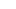# Time, Speed and Distance – All Formulas That You Need to Know

This triangle will help you remember the most basic formulae for TSD. Given below are a bunch of other concepts, formulae which come in handy while solving questions.

## Average and Relative Speed

If a body is moving with speeds s(1), s(2), s(3), … s(n) and the time is constant for each part, then the average speed will be the Arithmetic Mean of the speeds.

If a body is moving with speeds s(1), s(2), s(3), … s(n) and the distance is constant for each part, then the average speed will be the Harmonic Mean of the speeds.

Relative Speed of two bodies moving towards each other s(1)+s(2) and in the same direction s(1)-s(2).

## Boats, Streams and Escalators

If a constant distance is covered at speeds which are in an AP, then the times taken will be in an HP and vice-versa (For eg, Upstream, Boat-speed, Downstream speeds will always be in AP. Same can be applied in escalator questions as well).

Speed(Upstream) = S(Boat)-S(River)

Speed(Downstream) = S(Boat)+S(River)

Speed(Boat) = [S(Downstream)+S(Upstream)]/2

Speed(River) = [S(Downstream)-S(Upstream)]/2

## Motion of two bodies in a straight line

Two bodies start from opposite ends P & Q at the same time and move towards each other with speeds S(1) & S(2). After meeting each other, they take times of T(1) & T(2) to reach their destinations.

Time taken for them to meet sqrt[T(1)*T(2)]

S(1)/S(2) = sqrt[T(2)/T(1)]

Two bodies start from opposite ends P & Q at the same time and move towards each other with speeds S(1) & S(2). They reach the opposite ends and reverse directions. {S(1)>S(2) and S(1)

Total distance covered till nth meeting (2n-1)D and time taken (2n-1)D/S(1)+S(2).

## Circular MotionNumber of distinct points: In same direction, a-b and in opposite direction a+b (Here a/b is the reduced ratio of speeds).

I hope that with this set of formulae, you will be able to solve TSD questions with ease.

I will be conducting a workshop on TSD where I will be discussing this and other concepts.

## Enroll for Workshop

(Date: September 1 (Sunday). Time: 7:30 pm. Duration: 3 hours)

Ravi Handa, an alumnus of IIT Kharagpur, is the founder of HandaKaFunda. He teaches an Online CAT Coaching Course for CAT Preparation.